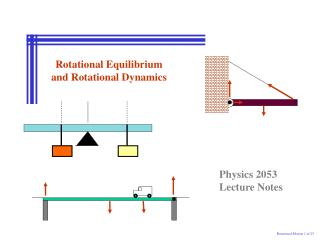DownloadDownload PresentationRotational Equilibrium and Rotational Dynamics

# Rotational Equilibrium and Rotational Dynamics

Télécharger la présentation## Rotational Equilibrium and Rotational Dynamics

- - - - - - - - - - - - - - - - - - - - - - - - - - - E N D - - - - - - - - - - - - - - - - - - - - - - - - - - -
##### Presentation Transcript

1. Physics 2053 Lecture Notes Rotational Equilibrium and Rotational Dynamics Rotational Motion 1 of 25

2. Rotational Equilibrium and Dynamics Topics 9-01 Torque 8-02 Torque and the Conditions for Equilibrium 8-03 Center of Gravity 8-04 Examples of Objects in Equilibrium Dynamics: Newton’s Laws of Motion

3. Torque r F Torque The force used to open a door produces a torque Static Equilibrium

4. Rotational Equilibrium Two equal forces are applied to a door. The first force is applied at the midpoint of the door; the second force is applied at the doorknob. Both forces are applied perpendicular to the door. Which force exerts the greater torque? A) the first at the midpoint B) both exert equal non-zero torques C) both exert zero torques D) the second at the doorknob Rotational Equilibrium

5. Torque r q F R Torque Static Equilibrium

6. Rotational Equilibrium Two equal forces are applied to a door at the doorknob. The first force is applied perpendicular to the door; the second force is applied at 30° to the plane of the door. Which force exerts the greater torque? A) the first applied perpendicular to the door B) the second applied at an angle C) both exert equal non-zero torques D) both exert zero torques Rotational Equilibrium

7. Torque and the Conditions for Equilibrium Conditions for Equilibrium SF = 0 St = 0 Static Equilibrium

8. Torque and the Conditions for Equilibrium N = ? 2nd Condition of Equilibrium 1st Condition of Equilibrium What is the Distance x2 What is the Normal Force A massless meter stick x3 = 20 cm x2 = ? o 3 kg 2 kg Static Equilibrium

9. Center of Gravity xcm y m2 m1 x cg x1 x2 Linear Momentum

10. Problem y x Find the center of gravity of the three-mass system shown in the diagram. Specify relative to the left-hand 1.00 kg mass. 1.0 kg 1.5 kg 1.1 kg 0.5 m 0.25 m Linear Momentum

11. Center of Gravity L L = 150 cm W = 600 N Wb= 50 N F = 200 N Find xcg Linear Momentum

12. Center of Gravity L N F L = 150 cm W = 600 N Wb= 50 N F = 200 N Linear Momentum

13. Examples of Objects in Equilibrium N1 N2 WB WT Truck on a Bridge 1st Condition 2st Condition Static Equilibrium

14. Examples of Objects in Equilibrium WT Truck on a Bridge N1 N2 WB 1st Condition 2nd Condition Static Equilibrium

15. Examples of Objects in Equilibrium T F N WB WS Physics Hanging a Sign q Static Equilibrium

16. Examples of Objects in Equilibrium Hanging a Sign d = L sin(q) Find Tension in cable d T F 1st Condition N q A L WB WS 2nd Condition Static Equilibrium

17. Examples of Objects in Equilibrium T F N WS WB d L How far (d) can a student walk on beam before cable breaks (Tmax) q Static Equilibrium

18. Examples of Objects in Equilibrium L d Tmax F q o N WS WB Walking Student When T reaches Tmax Static Equilibrium

19. END